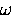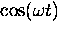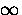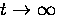# B Numerical Integration of a Highly Oscillatory Function

The complete program code that performs the numerical integration of Eq. (6.20) is given here. The evaluation of this integral is made complicated by the oscillatory nature of the integrand, and that we are integrating over bothand t in terms like. Fortunately, there is a natural upper cut-off in frequency given by the highest lattice modes (approximately the Debye frequency) but t still runs from to.

The key to integrating this function is to determine how far out in time one actually needs to integrate in order for the integral to converge. Since the integrand oscillates about zero and always decreases in amplitude as, the integral also oscillates as the upper limit in time is taken to infinity, and the range of these oscillations decreases as.This code decides when to stop integrating by comparing the range of oscillation of the integral to the mean of the last local minimum and maximum values of the integral. When the range of oscillation is a (pre-set) small fraction of the mean value, the value of the mean is taken to be the estimate of the integral in the limit.The algorithm requires the integrand to have its maximum value at t=0 and an envelope that decreases monotonically with time.

#define float double

#include <math.h>
#include <stdlib.h>
#include <stdio.h>
#include "1ph_hr_2.c"

void hop_rate_(double *, double *, double *, double *, double *);

void main()
{
float temperature, lp, lb,delta, hop_rate;
FILE *fp;

fp=fopen("1ph_hr_2d.out","w");

lp=38.801;
lb=2.5;
delta=0.079194;

fprintf(fp, " lp=%f \n", lp);
fprintf(fp, " lb=%f \n", lb);
fprintf(fp, " delta=%f \n", delta);

for(temperature=38; temperature<=116; temperature += 3.0){
hop_rate_(&temperature, &delta, &lb, &lp, &hop_rate);
fprintf(fp, " %E %E \n", temperature, hop_rate);
}

fclose(fp);
}


/*  1PH_HR_2.c  calculates hop rate in 1phonon model, with polaron
and flucuational barrier preparation by direct numerical integration.
Phonon spectrum of solid Xe is includedin function g(w).
*/

#define float double

#include <stdlib.h>
#include <stdio.h>
#include <math.h>

float my_int(float (*func)(float), float a, float b, long int n);
float  t_int(float (*func)(float));
float f1(float);
float f2(float);
float f1_approx(float);
float f2_approx(float);
float fg(float);
float g(float);
float f_of_t(float);
float f_of_t_test(float);
float f_approx(float);
float sqr(float);
float coth(float);
float hop_rate(void);
float f_lb(float);
float f_lp(float);
float sign(float);

/* global variables and constants used by several functions */
float t, tp, omega, omega_d, th_d, lb, lp, delta, gf;
float hbar, kb, ff;
float bb_fctr, bb_om;
long int nn;

void hop_rate_(double *ptp, double *pdelta, double *plb, double *plp,
double *hpr)
{
float time_range, t1, t2, dt, dtime, tt, foft, z1,z2;
float i1,i2;
long int n_cur, curve, nn_max;

hbar = 1.055E-34;            /* Plank's constant                    */
kb = 1.381E-23;              /* Boltzman's constant                 */
ff = hbar/kb;
th_d=64.0;                   /* Debye temperatrue of Xe */
omega_d = kb*th_d/hbar;

tp=*ptp;
lb=*plb;
lp=*plp;
delta=*pdelta;

nn=4000;
if(tp>=110) nn=8000;
gf= my_int(*fg, 0.0, omega_d, 8000);
z1=2.0*t_int(*f_of_t);
*hpr=sqr(delta*kb/hbar)*z1;
return;
}

/* t_int integrates an oscillatory function of time, whose  amplitude
decreases with time,  from zero up to such time that the amplitude of
oscillation of its integral is small compared to the mean, which is taken to be
the limiting value of the integral. I.e., it goes as far out as it needs
to so that it is clear to what value it is converging */

#define FUNC(x) ((*func)(x))
float t_int(float (*func)(float))
{
float a,sum, del, m, maxval, minval,cc;
int goodmax, goodmin,conv=0;

del=4.0E-17;  /* fixed step size in seconds */
cc=1.0E-2;    /* Convergence criteria: (max-min) < 0.01*mean */
maxval=0.0;
minval=0.0;

sum=0.0E0;
a=del/2.0;    /* start offset at centre of first interval */
m=0.0E0;
while(!conv){
goodmax=0;
goodmin=0;
while(!goodmax){
sum += FUNC(a + m*del);
m++;
if(sum<=maxval) goodmax=1;    /* found new maxval */
else maxval=sum;
}

minval=maxval;
while(!goodmin){
sum += FUNC(a + m*del);
m++;
if(sum>=minval) goodmin=1;    /* found new minval */
else minval=sum;
}

if( (maxval-minval)< cc*(maxval+minval)*0.5) conv=1;
else{
goodmax=0;
goodmin=0;
maxval=minval;
}
}
return((maxval+minval)*0.5*del);
}
#undef FUNC(x)

/* My dumb, brute-force integrator */
#define FUNC(x) ((*func)(x))
float my_int(float (*func)(float), float a, float b, long int n)
{
float x, sum, del, m;

del=(b-a)/n;
sum=0.0;
a=a+del/2.0;
for (m=0; m<=n-1; m++){
sum += FUNC(a + m*del);
}
return(sum*del);
}
#undef FUNC(x)

float f_of_t(float x)     /* the function of time that gets integrated */
{
float f, psi_bar, psi;

t=x;    /* passes time to global variable t */

psi_bar = my_int(*f1, 0.0, omega_d, nn);
psi     = my_int(*f2, 0.0, omega_d, nn);

f=exp(psi_bar+gf)*cos(-psi);  /* im part = sin(psi) dropped */

return(f);
}

float f1(float w)     /* integrand for Psi bar - fcn of omega */
{
float l_fctr, v;

if(w==0.0) return(0.0);

l_fctr=(lb*hbar*w)/(kb*th_d) + lp*kb*th_d/(hbar*w);
v=g(w)*l_fctr*cos(w*t)/sinh(hbar*w/(2.0*kb*tp));
return(v);
}

float f2(float w)      /* integrand for Psi - fcn of omega    */
{
float l_f,v;

if(w==0.0) return(0.0);
l_f = 2.0*sqrt(lb*lp);
v=l_f * g(w) * sin(w*t)/sinh((hbar*w)/(2.0*kb*tp));

return(v);
}

float fg(float w)
{
float tmp,v;

if(w==0.0) return(0.0);
tmp=(lb*hbar*w)/(kb*th_d) - lp*(kb*th_d)/(hbar*w);
v=g(w)*tmp*coth((hbar*w)/(2.0*kb*tp));
return(v);
}

/* Real g(w) for s-Xe by simple linear interpolation between points*/
/* Data read from a graph in Klein's Rare Gas Solids , p964 ? */
float g(float omega)
{
float  x, y, t_dist;
long int i;

float yy =
{0.0,0.07,0.15,0.30,0.45, .6,.9,1.1,1.32,1.7,
2.0,2.2,2.7,3.1,3.8, 4.25,5.0,5.7,6.3,7.1,
8.0,9.3,10.6,11.7,13.0, 14.8,16.5,18.6,21.0,24.5,
34.0,34.93,35.86,36.77,37.71, 38.64,39.57,40.50,41.43,42.36,
43.29,44.21,45.14,46.07,47.00, 28.0,27.9,27.8,27.6,27.2,
27.0,26.0,25.0,23.0,21.0, 18.5,36.0,43.0,51.0,
64.0,33.0,22.0,10.0,0.0,0.0,0.0,0.0,0.0};

x=omega*(97.9/12.57E12);       /* scale interpolation variable */
i=x;
if(x<=10){
y= 0.019*x*x;                  /* at low omega like omega^2 */
return(y/1.7217424E14);
}
if(i>=64){                        /* above cutoff -> zero      */
y=0.0;
}
else{
y=yy[i] + (x - (float) i)*(yy[i+1] - yy[i]);
}
return(y/1.7217424E14);   /* normalized so its integral is 1.0000 */
}

/* def some math */
float sqr(float x)
{
return(x*x);
}

float coth(float x)
{
return(cosh(x)/sinh(x));
}